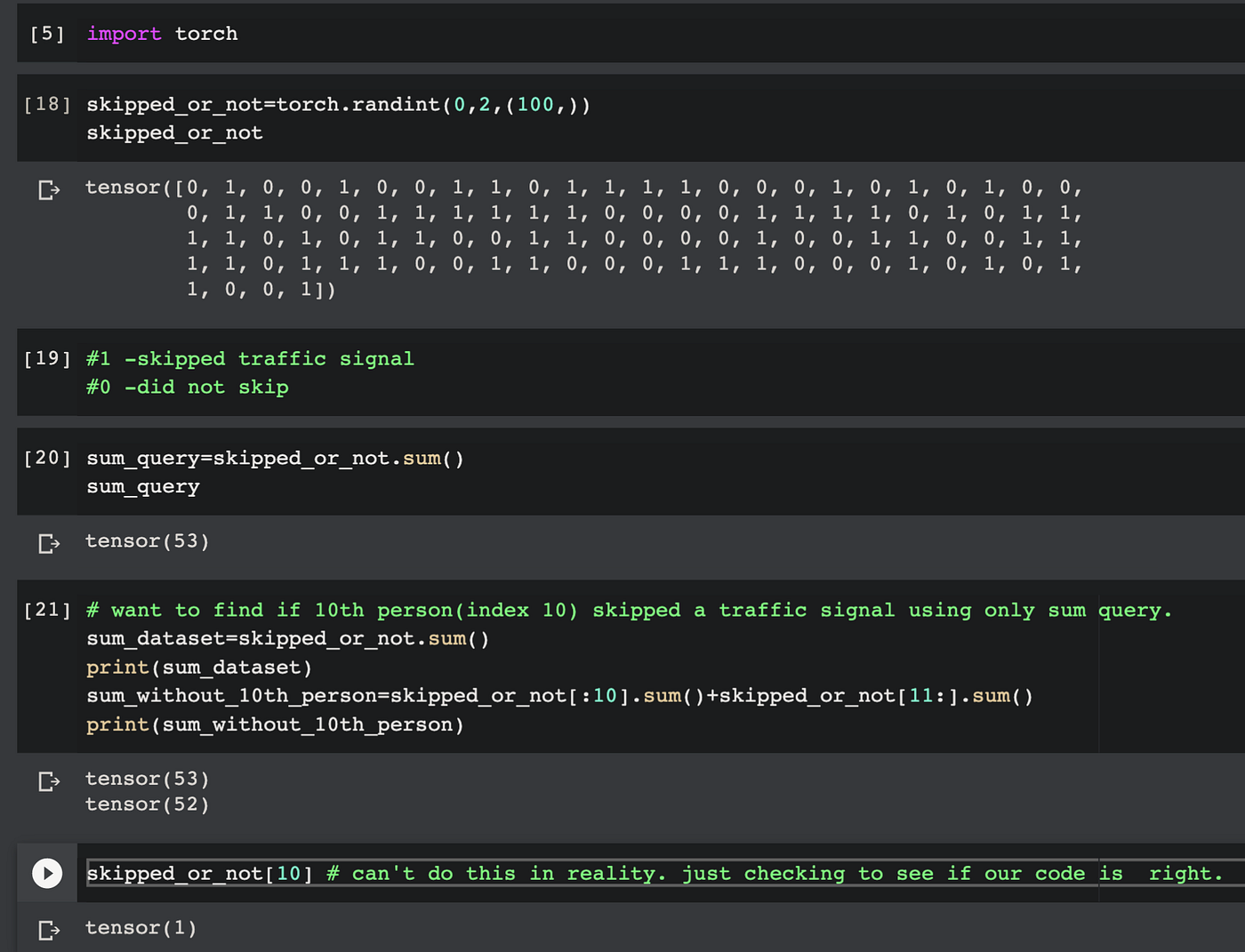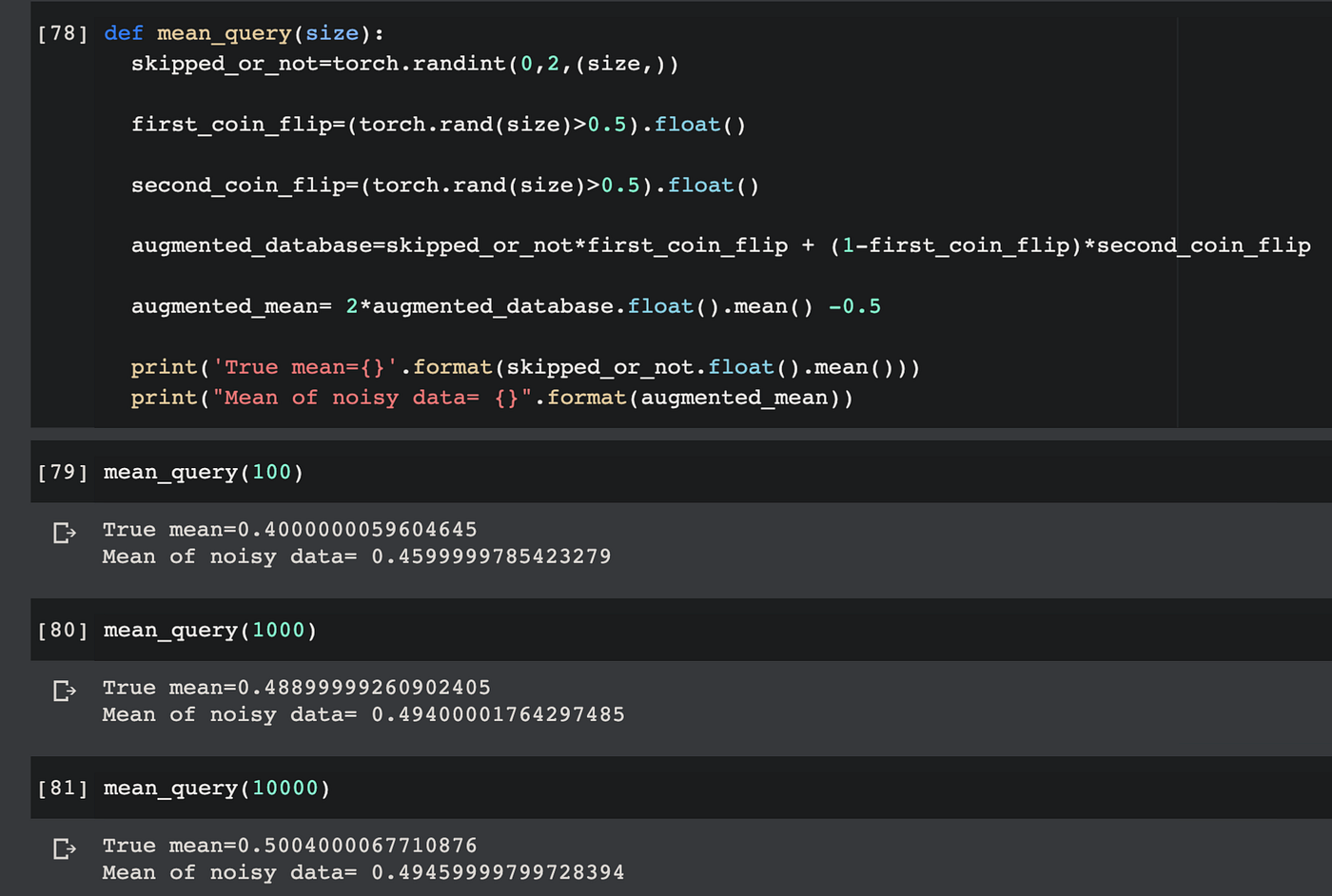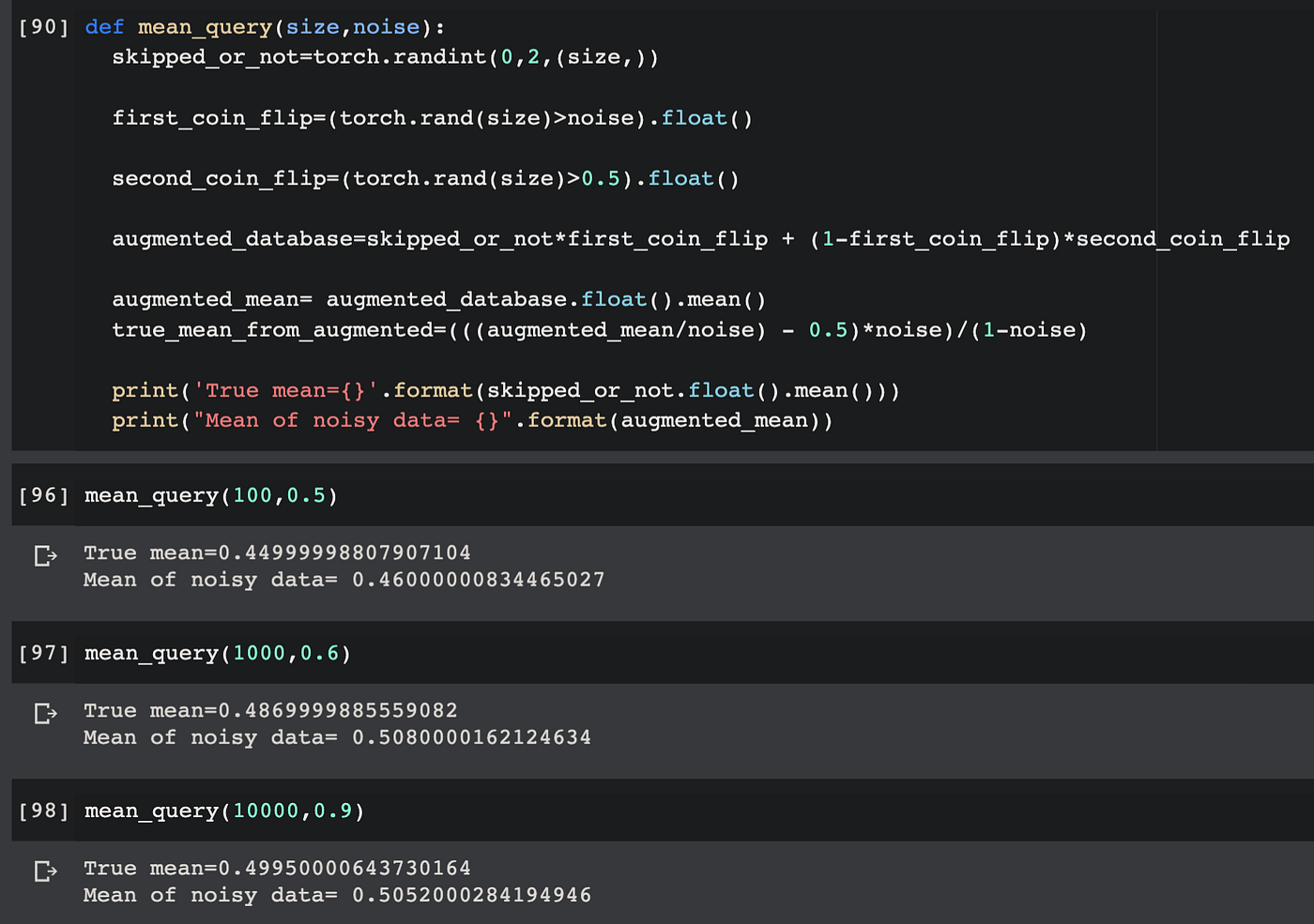# How your data is secured(by a coin toss)

## An introductory piece regarding localised differential privacy.Photo by Dayne Topkin on Unsplash

Assume you want to survey a population of 1000 and ask them if they’ve skipped past a traffic signal. The data that you are collecting here is sensitive. Assume you want to build a dataset regarding human behaviour to perform some sort of analysis. ‘Skipped Traffic Signal’ will be one column of this dataset.

Now you, the curator of the dataset want to make this data public so that someone can perform some statistical analysis on it. The analyst can then query the ‘Skipped Traffic Signal’ column of the dataset to find out if a particular person skipped the signal or not. But the people in the dataset want their data to be secured. They don’t want an analyst to be able to query something about them and be a 100 percent sure of their activities.

The question now is, how do we ensure the privacy of every individual while also providing the analyst data that is not very noisy so that he/she can draw value from it?

We can conduct a simple experiment to do so:

Response Yes(1)= Skipped traffic signal

Response No(0)=Didn’t do so.

1. Toss a coin once. If it lands on heads the individual has to tell the truth(whether he/she skipped a traffic signal or not).
2. If the first coin lands on tails, toss the coin again. Now the individual has to answer ‘Yes’ if it lands on heads and ‘No’ if it lands on tails. We aren’t giving the person really a choice here. This is where we add ‘randomness’.

Think about the probability of a person responding ‘Yes’ even though that person actually hasn’t skipped a traffic signal.

For this to happen, the second coin toss has to occur and it has to land on heads. That’s because if the first toss was heads, the person would’ve told that they did not skip the signal. But if the first coin toss is tails and the second coin toss is heads the person will respond Yes even though the true response is No.

# Some basic probability:

P(Yes|No)=P(tails on first toss)*P(heads on second toss)=0.5*0.5=0.25

What is the probability of a person responding Yes when the true answers is in fact Yes?

This can happen in two ways. If the first toss is heads they have to say the truth which is Yes. Or the first toss can end up being tails and the second heads.

P(Yes|Yes)=P(heads on first toss) + P(tails on first toss)*P(heads on second toss)=0.5 + 0.5*0.5 =0.75.

Here P(A|B) is a conditional probability meaning the probability of A happening given B has already happened. If you want to know more about probabilities you can check out Khan academy’s course.

Now imagine that the ‘True proportion’ of people who skipped a traffic signal is ‘p’. And those who did not is naturally ‘1-p’. What are the expected number of “Yes” answers once the coin toss experiment is done?

Again for this look back at the two probabilities, we computed. Yes can happen in two ways. Either the person skipped the traffic signal and responded Yes(P(Yes|Yes)) or he did not and responded Yes(P(Yes|No)).

A. Out of the proportion 1-p who did not skip the traffic signal how many people are expected to say “Yes”?.

B. Out of the proportion “p” who did skip the traffic signal how many people are expected to say “Yes”?.

If we add up these two expected “Yes” values we get the expected number of “yes” in the whole population.

This is the formal definition of expected value. If we translate it to our case it would look something like this:

E(Yes)=(1-p)*P(Yes|No) + p*P(Yes|Yes)

If you look at the individual components closely they answer questions A and B respectively. So E(Yes)=(1-p)*0.25 + p*0.75.

But the true value of E(Yes) =p.

Hence we have added “noise” to our dataset. As explained in Cynthia Dwork’s book on “The Algorithmic Foundations of Differential Privacy” the coin toss gives people plausible deniability. That means even if the coin toss ends up in them answering Yes even though the true answer is No they can deny it and blame it on the coin toss(the second toss to be specific as it is in this toss we don’t really give the person to tell the truth. It’s random.).

Now imagine you are an analyst who wants to look at this data of people who tossed the coin. Let us say you want to query from the dataset a particular person to find whether he/she skipped a traffic signal. Imagine what this would look like if the data had not been private by the coin toss.

In the above representation it’s made clear that the analyst can’t directly access the database. He/she can only query the database to a guard. This guard gets data from the database, adds appropriate amount of noise to it and sends back the noisy answer to the user.

# Let us make this more concrete with code.Here the analyst can only use queries like the sum query. Therefore, if the analyst let’s say wants to find out if the 10th person skipped the signal or not, he could just run a sum query on the entire database and another sum query without the 10th index. If both these sums are same, that means the value at the 10th index was 0. Or else the value was 1. Privacy is breached in this case. But if we had added noise to the data, then the sum query can’t breach privacy. The analyst can’t really be sure of whether a particular value is 1 or 0.

Now let us add noise to the dataset so the analyst can’t find out information about a particular user.

The following code is also discussed in a video on Udacity which is a part of a differential privacy course.In the above code, the augmented database is obtained after the 2 coin flips. The interesting thing happening here is that we are multiplying the augmented mean by 2 and subtracting 0.5 from it. Why is that?

Again look back at the formula we derived for the expected number of “Yes” after the 2 coin flips are done on everyone. It was :

E(Yes)=(1-p)*0.25 + p*0.75=1/4 -p/4 +3p/4=1/4- p/2.

Now this expected value is not a true representation of the population. Even though we are adding privacy to users we cannot report this mean back to the analyst. We would still want the analyst to obtain valuable information about the dataset while at the same time preserving privacy of all users.

So essentially we need to get back “p” from 1/4 -p/2. This is done by multiplying the whole thing by 2 and subtracting 0.5 from it.

From the code above we find that having more data makes it easier for us to report an accurate representation of the population while still preserving privacy of the user. The true mean and the mean of noisy data converge as data points increase. Now we can also try to bias the coin to add more noise.

Let us say we bias only the first coin by making probability of tails=noise where noise is a value between 0 and 1.

Look at how the probabilities change:

P(Yes|No)=P(tails on first toss)*P(heads on second toss)=(noise)*0.5

P(Yes|Yes)=P(heads on first toss) +P(tails on first toss)*P(head on second toss) = (1-noise)+noise*0.5

E(Yes)=(noise)*0.5*(1-p) + (1-noise +noise*0.5)*p. This is similar to what we did previously. I have just replaced P(heads on first toss) with t.

Thus after simplifying the equation we get:

E(Yes)(noisy)= noise*0.5 +(1-noise)*p. This is the expected value of augmented data.

After some rearrangement:

True E(Yes) =(E(Yes)/noise-0.5)*(noise/(1-noise))=p

Let us look at this in code:Here in the first coin toss we have modelled it as torch.rand(size)>noise. This means we assign a value 1 only if it is greater than noise. Or in other words, we are assigning 1 (1-noise) proportion of times which is nothing but the probability of heads. Look at the last example where we have 10,000 data points and add a noise of 0.9! This ensures high privacy of users.

But wait, our estimate of the true mean is also very close to the actual mean “p” in the case where 10,000 data points are present. This means that we can afford to add a lot of noise if there are a lot of data points and still preserve accuracy of reported data.

Hence Local Differential Privacy works better when there is more data. This might sound a bit counterintuitive but it makes sense as we have more data noises tend to cancel each other out and we can get a better representation of the population.

I hope this is a good introduction on Differential Privacy. Will see you soon!

References:

1. An implementation of local DP without adding noise.

2. An implementation of local DP after adding noise.

3. Khan academy’s course on Probability.

4. The link to “The Algorithmic Foundations of Differential Privacy” by Cynthia Dwork and Aaron Roth. This is the book to dig deep into differential privacy.

.https://www.cis.upenn.edu/~aaroth/Papers/privacybook.pdf